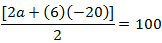Guru

# A sum of Rs 700 is to be used to give seven cash prizes to students of a school for their overall academic performance. If each prize is Rs 20 less than its preceding prize, find the value of each of the prizes. Q.16

• 0

The best question for class 10th of arithmetic progressions of exercise 5.3 of math . How i solve this question because it is very tough question A sum of Rs 700 is to be used to give seven cash prizes to students of a school for their overall academic performance. If each prize is Rs 20 less than its preceding prize, find the value of each of the prizes.

Share

1. Let the cost of 1st prize be Rs. P.

Cost of 2nd prize = Rs. P − 20

And cost of 3rd prize = Rs. P − 40

We can see that the cost of these prizes are in the form of A.P., having common difference as −20 and first term as P.

Thus, a = P and d = −20

Given that, S7 = 700

By the formula of sum of nth term, we know,

Sn = n/2 [2a + (n – 1)d]

7/2 [2a + (7 – 1)d] = 700a + 3(−20) = 100

a −60 = 100

a = 160

Therefore, the value of each of the prizes was Rs 160, Rs 140, Rs 120, Rs 100, Rs 80, Rs 60, and Rs 40.

• 0